# Resources tagged with: Square numbers

Filter by: Content type:
Age range:
Challenge level:

### There are 24 results

Broad Topics > Numbers and the Number System > Square numbers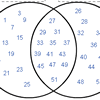### Various Venns

##### Age 7 to 11 Challenge Level:

Use the interactivities to complete these Venn diagrams.### Odd Squares

##### Age 7 to 11 Challenge Level:

Think of a number, square it and subtract your starting number. Is the number you’re left with odd or even? How do the images help to explain this?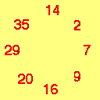### Cycling Squares

##### Age 7 to 11 Challenge Level:

Can you make a cycle of pairs that add to make a square number using all the numbers in the box below, once and once only?### Always, Sometimes or Never? Number

##### Age 7 to 11 Challenge Level:

Are these statements always true, sometimes true or never true?### Light the Lights Again

##### Age 7 to 11 Challenge Level:

Each light in this interactivity turns on according to a rule. What happens when you enter different numbers? Can you find the smallest number that lights up all four lights?### Sticky Numbers

##### Age 11 to 14 Challenge Level:

Can you arrange the numbers 1 to 17 in a row so that each adjacent pair adds up to a square number?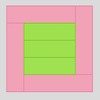### Cuisenaire Squares

##### Age 7 to 11 Challenge Level:

These squares have been made from Cuisenaire rods. Can you describe the pattern? What would the next square look like?### Two Primes Make One Square

##### Age 7 to 11 Challenge Level:

Can you make square numbers by adding two prime numbers together?### Swimming Pool Tiles

##### Age 7 to 11 Challenge Level:

This activity creates an opportunity to explore all kinds of number-related patterns.### One Wasn't Square

##### Age 7 to 11 Challenge Level:

Mrs Morgan, the class's teacher, pinned numbers onto the backs of three children. Use the information to find out what the three numbers were.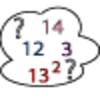### Seven Square Numbers

##### Age 7 to 11 Challenge Level:

Add the sum of the squares of four numbers between 10 and 20 to the sum of the squares of three numbers less than 6 to make the square of another, larger, number.### Augustus' Age

##### Age 7 to 11 Challenge Level:

In 1871 a mathematician called Augustus De Morgan died. De Morgan made a puzzling statement about his age. Can you discover which year De Morgan was born in?### Picture a Pyramid ...

##### Age 7 to 11 Challenge Level:

Imagine a pyramid which is built in square layers of small cubes. If we number the cubes from the top, starting with 1, can you picture which cubes are directly below this first cube?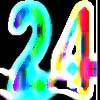### Special 24

##### Age 7 to 11 Challenge Level:

Find another number that is one short of a square number and when you double it and add 1, the result is also a square number.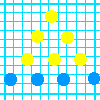### Graphing Number Patterns

##### Age 7 to 11 Challenge Level:

Does a graph of the triangular numbers cross a graph of the six times table? If so, where? Will a graph of the square numbers cross the times table too?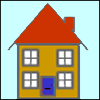### A Right Charlie

##### Age 7 to 11 Challenge Level:

Can you use this information to work out Charlie's house number?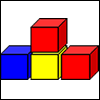### Up and Down Staircases

##### Age 7 to 11 Challenge Level:

One block is needed to make an up-and-down staircase, with one step up and one step down. How many blocks would be needed to build an up-and-down staircase with 5 steps up and 5 steps down?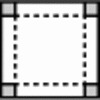### Making Boxes

##### Age 7 to 11 Challenge Level:

Cut differently-sized square corners from a square piece of paper to make boxes without lids. Do they all have the same volume?### Factors and Multiples Puzzle

##### Age 11 to 14 Challenge Level:

Using your knowledge of the properties of numbers, can you fill all the squares on the board?### Fractions in a Box

##### Age 7 to 11 Challenge Level:

The discs for this game are kept in a flat square box with a square hole for each. Use the information to find out how many discs of each colour there are in the box.### A Square Deal

##### Age 7 to 11 Challenge Level:

Complete the magic square using the numbers 1 to 25 once each. Each row, column and diagonal adds up to 65.### Digat

##### Age 11 to 14 Challenge Level:

What is the value of the digit A in the sum below: [3(230 + A)]^2 = 49280A### Square Routes

##### Age 11 to 14 Challenge Level:

How many four digit square numbers are composed of even numerals? What four digit square numbers can be reversed and become the square of another number?### Time of Birth

##### Age 11 to 14 Challenge Level:

A woman was born in a year that was a square number, lived a square number of years and died in a year that was also a square number. When was she born?